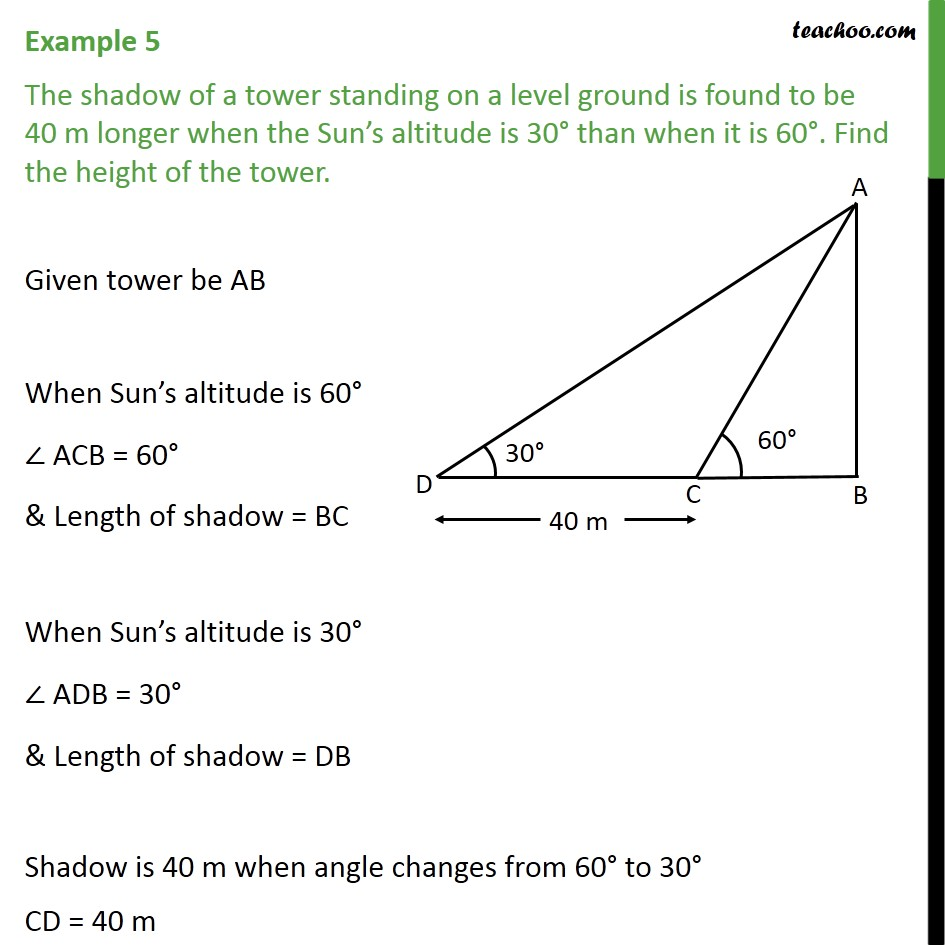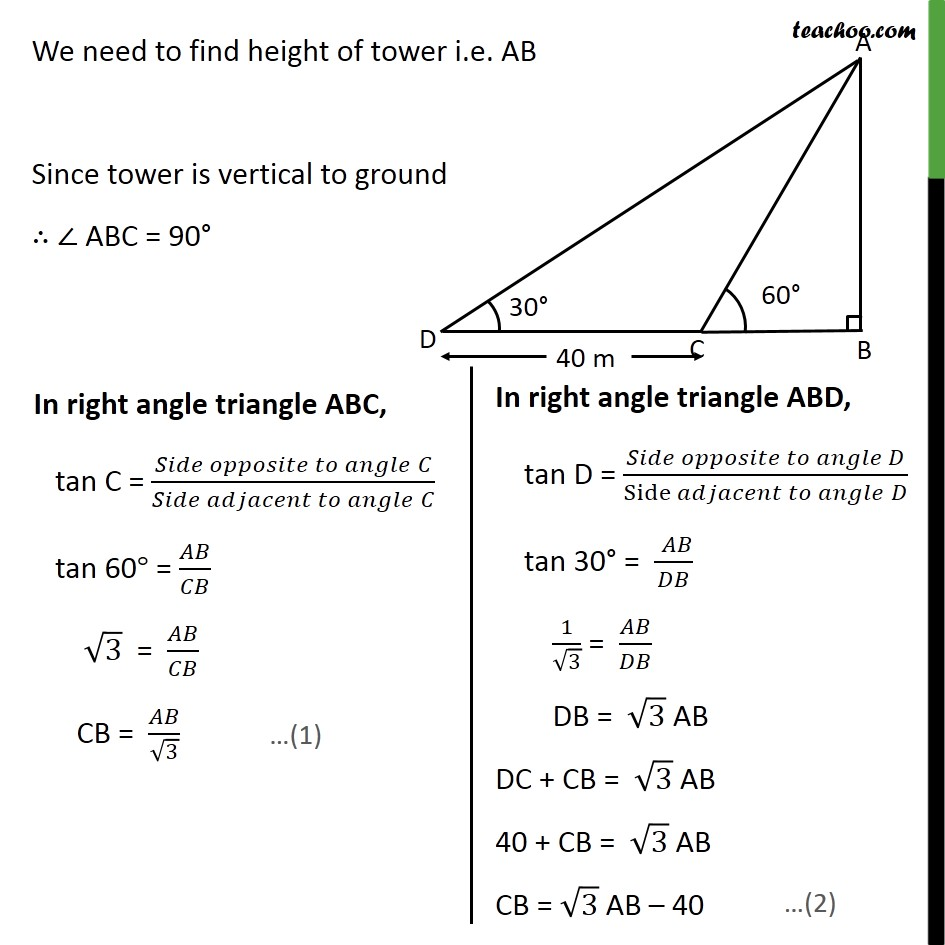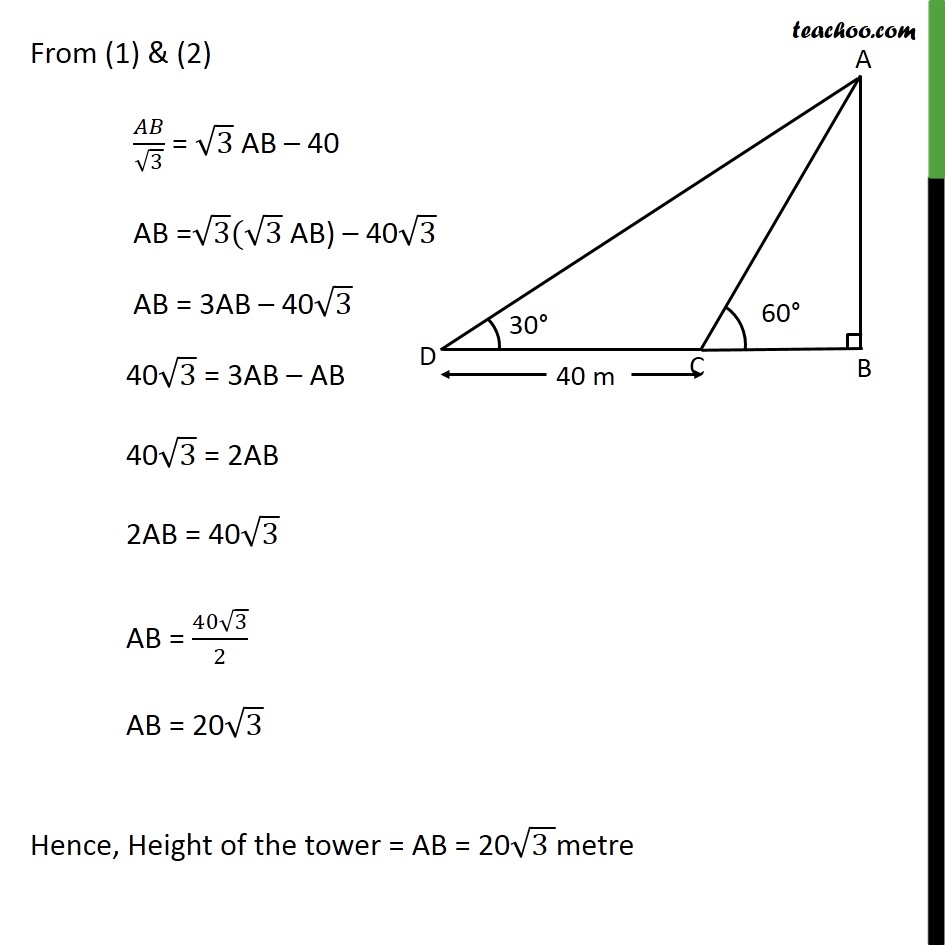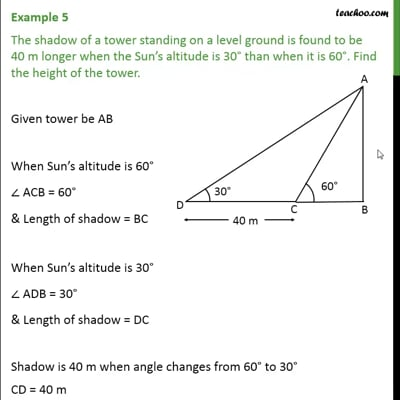Examples

Chapter 9 Class 10 Some Applications of Trigonometry
Serial order wiseThis video is only available for Teachoo black users

Maths Crash Course - Live lectures + all videos + Real time Doubt solving!

### Transcript

Example 5 The shadow of a tower standing on a level ground is found to be 40 m longer when the Sun’s altitude is 30° than when it is 60°. Find the height of the tower. Given tower be AB When Sun’s altitude is 60° ∠ ACB = 60° & Length of shadow = BC When Sun’s altitude is 30° ∠ ADB = 30° & Length of shadow = DB Shadow is 40 m when angle changes from 60° to 30° CD = 40 m We need to find height of tower i.e. AB Since tower is vertical to ground ∴ ∠ ABC = 90° From (1) & (2) 𝐴𝐵/√3 = √3 AB – 40 AB" =" √3(√3 " AB) – 40" √3 AB = 3AB –" 40" √3 "40" √3 = 3AB – AB "40" √3 = 2AB 2AB = "40" √3 AB = (40√3)/2 AB = 20√3 Hence, Height of the tower = AB = 20√(3 )metre